Get instant live expert help with Excel or Google Sheets“My Excelchat expert helped me in less than 20 minutes, saving me what would have been 5 hours of work!”

#### Post your problem and you’ll get Expert help in seconds.

Your message must be at least 40 characters
Our professional Expert are available now. Your privacy is guaranteed.

# How to use the Decimal Function in Excel – Excelchat

The Excel DECIMAL function allows us to convert alpha-numeric representations of numbers in a specified base into their equivalent in decimals.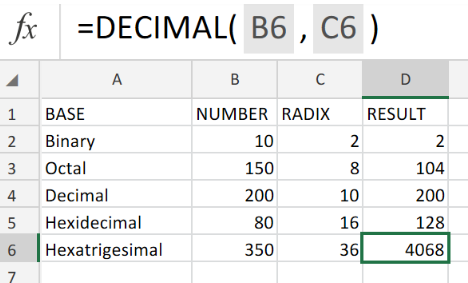Figure 1. of Excel Decimal Function

## Generic Formula

`=DECIMAL (number, radix)`

• number = alpha-numeric values
• radix – A number value representing the base of the number to be converted (within the range of 2 – 36)

## How to Set Decimal Places in Excel

1. First, we have to set up the available data in our Excel worksheet: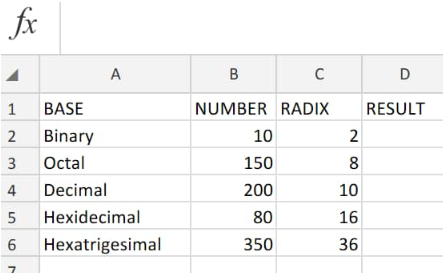Figure 2. of Decimal Function in Excel

The input string of numbers (column B above) must contain only valid alpha-numeric characters which correspond with our supplied base (radix).

Below is a standard list of alpha-numeric characters with their correspond base values: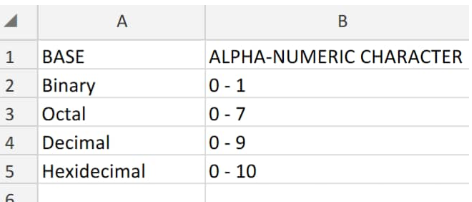Figure 3. of Standard Alpha-numeric and Base Values in Excel

Our goal in Figure 2. above, is to convert the NUMBER values in column B into the  decimal Excel values of the five different bases in column A.

1. The Decimal Excel formula we will enter into cell D2 of our worksheet example is as follows:

`=DECIMAL(B2,C2)`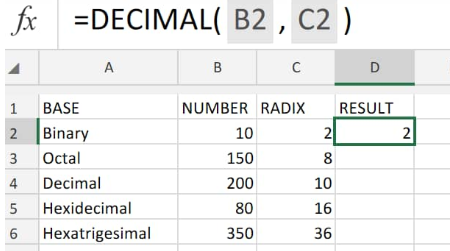Figure 4. of Decimal Function in Excel

The number in cell B2 is a binary value that has a base 2.  We now know its Excel decimal value is 2 (cell D2).

1. To determine the succeeding Excel Decimal values, modify and copy Excel Decimal formula in D2 down into other cells in the column: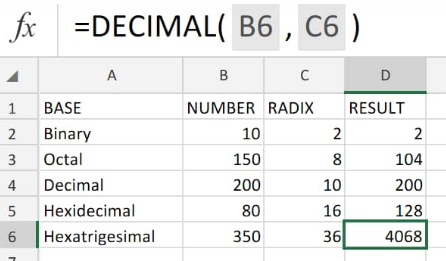Figure 5. of Decimal Function in Excel

Each number is then converted its corresponding Excel decimal value in cells D4to D6.

## How to Adjust Decimal Places in Excel

If our goal is to specify or move decimal points in Excel, follow the simple steps below:

2. Click on the “Format Cells” button, and then a “Format Cells” window will emerge;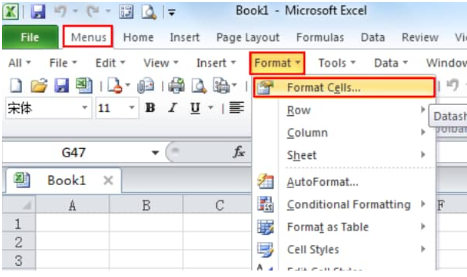Figure 6. of Format Cells Button in Excel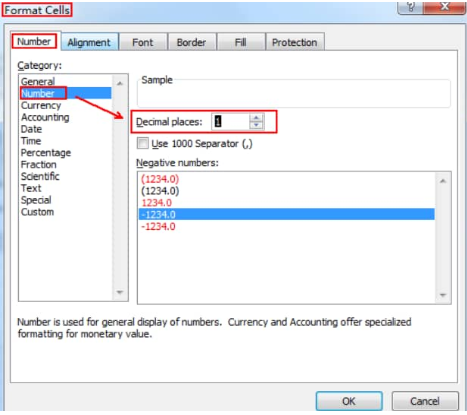Figure 7. of Format Cells Window in Excel

1. Click on the “Number” tab, and then select the “Number” item from the Category list; we should locate the “Decimal Place” button in the right section of the window. From here, we can now format the exact number of decimal places in Excel.

## How to Limit Decimal Places in Excel

We can easily limit decimal places in Excel by formatting cells.

Follow the steps below to make Excel limit decimal places

1. Select the cell(s) which we want to change their decimal Excel limit and then right click on those selected cells, before selecting the “Format Cells” option which is on the right-click menu;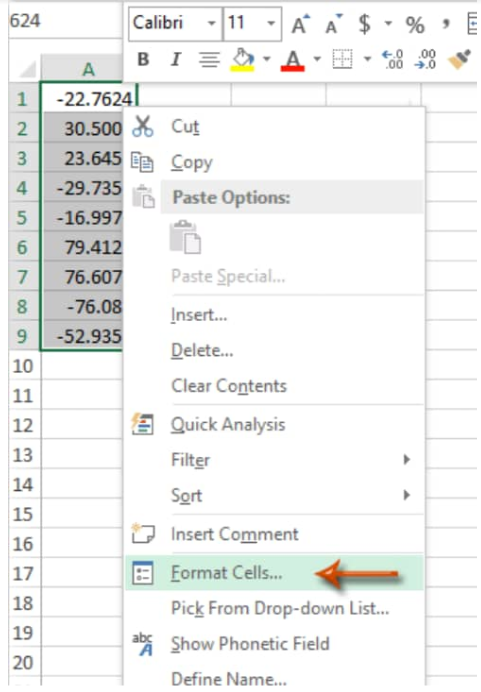Figure 8. of Format Cells Button in Excel

1. In the next “Format Cells” window, click on the “Number” tab, and then choose “Number” in the Category section, and then enter a number value representing the decimal limit inside the “Decimal Places”input bar..

For example, if we seek to limit the selected cells to just 1 Excel decimal place, simply type “1”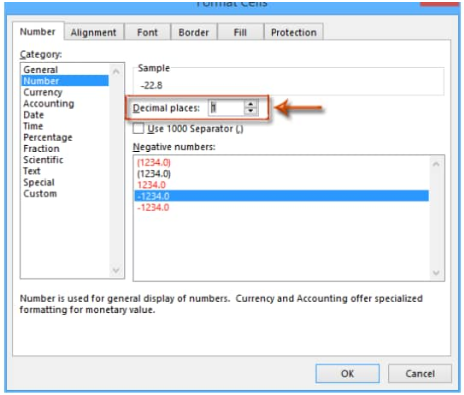Figure 9. of Decimal Limit in Excel

1. Clicking on “OK” will close the Format Cells window and then you will observe all the decimals in our selected cells have been changed to a single Excel decimal place.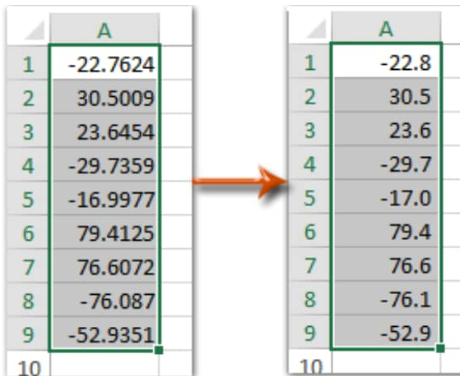Figure 10. of Limit Decimal Places in Excel